## Indicators

•Cited by SciELO
•Access statistics

•Similars in SciELO

## Print version ISSN 0187-6236

### Atmósfera vol.25 n.3 México Jul. 2012

Frictional convergence, atmospheric convection, and causality

D. J. Raymond and M. J. Herman

Physics Department and Geophysical Research Center, New Mexico Tech, Socorro, NM, USA. Corresponding author: D. J. Raymond; e-mail: raymond@kestrel.nmt.edu

Received August 12, 2011; accepted June 24, 2012

RESUMEN

En este trabajo se considera la convergencia por fricción en una capa límite atmosférica acotada por una troposfera estable libre. De acuerdo con un extenso trabajo previo encontramos que la estabilidad atmosférica reduce la escala vertical de la circulación troposférica secundaria libre asociada con la convergencia por fricción. Relacionada con esta reducción en la escala vertical hay una reducción proporcional en la escala temporal debido a la fricción por el giro descendente de la circulación atmosférica. Esta reducción en la escala temporal modifica el balance entre términos del componente en la ecuación de momento a lo largo de las isobaras. En particular, para las escalas de perturbación menores a unos cuantos cientos de kilómetros en condiciones tropicales típicas, la tendencia del término de momento llega a un balance aproximado con el término de fricción, haciendo que el término de Coriolis sea menos importante. Esto reduce la magnitud del flujo isobárico cruzado y la fuerza ascendente en las regiones donde este flujo converge. Si algún otro mecanismo, tal como la convergencia húmeda, produce suficiente convergencia de capa límite para anular el giro descendente de la perturbación en cuestión, entonces la magnitud de la convergencia iguala a la predicha por la fórmula de la convergencia por fricción de estado estable. Sin embargo, en este caso la flecha de la causalidad es invertida en comparación con la que se asume en el tratamiento simple de la convergencia por fricción. La convergencia por fricción no "causa" la convección, realmente la convección causa la convergencia y el mecanismo que fuerza la convección debe buscarse en otro lado. Esta distinción es crucial para entender qué produce la convección profunda. El presente análisis es lineal y la perspectiva puede cambiar cuando los efectos no lineales son importantes. También está limitado a situaciones en las que los vientos de la capa limite son relativamente débiles. Por lo tanto, los ciclones tropicales con sus fuertes vientos y comportamiento no lineal merecen un análisis independiente.

ABSTRACT

Frictional convergence in an atmospheric boundary layer topped by a stable free troposphere is considered. In agreement with extensive previous work, we find that atmospheric stability reduces the vertical scale of the free tropospheric secondary circulation associated with frictional convergence. Associated with this reduction in vertical scale is a proportional reduction in the time scale for the frictional spindown of an atmospheric circulation. This reduction in time scale alters the balance between terms in the component of the momentum equation along the isobars. In particular, for disturbance scales less than a few hundred kilometers in typical tropical conditions, the momentum tendency term comes into approximate balance with the friction term, with the Coriolis term becoming less important. This reduces the magnitude of the cross-isobaric flow and the strength of the ascent in regions where this flow converges. If some other mechanism such as moist convection produces enough boundary layer convergence to nullify the spindown of the disturbance in question, then the magnitude of the convergence equals that predicted by the steady-state frictional convergence formulation. However, in this case the arrow of causality is reversed from that assumed in a naive treatment of frictional convergence.

Frictional convergence is not "causing" the convection; the convection is actually causing the convergence, and the mechanism forcing the convection must be sought elsewhere. This distinction is crucial in understanding what drives deep convection. The present analysis is linearized and the picture may change when nonlinear effects become important. It is also limited to situations in which the boundary layer winds are relatively weak. Tropical cyclones, with their strong winds and nonlinear behavior, thus deserve an independent analysis.

Keywords: Atmospheric stability, frictional spindown, momentum equation, tropical conditions.

1. Introduction

The frictional spindown of a rotating fluid over a stationary surface is a classical problem in geophysical fluid dynamics, first considered by Ekman (1905, 1906; see also Duck and Foster, 2001). In this scenario, cross-isobaric flow is induced in the boundary layer by friction as part of a deep secondary circulation which spins down the entire fluid. Charney and Eliassen (1949) proposed that frictionally induced cross-isobaric flow produces upward motion at the top of the boundary layer when this flow is convergent. (They further noted that Albert Einstein earlier employed this idea to explain the clustering of tea leaves in the center of a stirred cup of tea in his book Mein Weltbild). This idea later became an integral part of their theory of tropical cyclogenesis (Charney and Eliassen, 1964) and in Charney's ideas about the forcing of convection in the intertropical convergence zone (ITCZ; Charney 1971) and tropical cloud clusters (Charney, 1973).

That frictionally induced convergence of boundary layer air and the resulting ascent at the top of the boundary layer force convection in the tropics has become a staple of tropical meteorology. Ooyama's (1969) model of the development of a tropical cyclone incorporated this mechanism as have models of large-scale tropical waves (e. g., Holton et al1971; Holton, 1974; Wang, 1988; Wang and Rui, 1990).

Holton (1965) investigated the role of stratification of a rotating fluid in the spindown problem. In an unstratified fluid, the secondary circulation associated with frictionally induced cross-isobaric flow in the boundary layer extends through the full depth of the fluid. If the fluid depth is much greater than the boundary layer depth, then the time scale for spindown is equal to the rotational time scale times the ratio of fluid depth to boundary layer depth (Greenspan and Howard, 1963). When this ratio is large, the time scale for spindown is much greater than the rotational time scale, which allows the approximation of time-independence to be made in the calculation of cross-isobaric flow in the boundary layer. However, if the fluid is stratified, then the secondary circulation is confined to a shallower layer, and the spindown time decreases in comparison to the unstratified case.

Significant controversy surrounded Holton's (1965) paper shortly after it appeared (Pedlosky, 1967; Holton and Stone, 1968; Sakurai, 1969; Duck and Foster, 2001). However, the controversy had to do primarily with Holton's treatment of the side wall in laboratory experiments. As we are interested in the atmosphere where side walls do not exist, we ignore this controversy; the central result that spindown is confined to a shallow layer and consequently occurs more rapidly in the presence of stratification has not been challenged.

In the case of strong stratification, the spindown time may be short enough that the assumption of time-independence in the boundary layer calculation of cross-isobaric flow is no longer justified. Holton et al. (1971) and Holton (1974) include time dependence explicitly in their analyses of the boundary layer. However, many authors do not. The purpose of this paper is to highlight the potential importance of this issue, especially as it applies to the interaction between frictionally induced flow and deep atmospheric convection. In particular, we address the question as to whether boundary layer convergence "causes" convection or whether the convection "causes" the convergence. This question is important for understanding the dynamics of tropical, convectively coupled disturbances such as ITCZs, tropical waves, tropical cyclones, and even the Madden-Julian oscillation.

In section 2 the response of a uniformly stratified atmosphere to surface friction is reviewed in a simple, linearized context. The analysis is extended to a neutral boundary layer topped by a stably stratified atmosphere in section 3. Section 4 discusses the interaction between frictional convergence and convection in these cases, and conclusions are presented in section 5.

2. Stratified spindown

For purposes of exposition, in this section we postulate a very simple atmosphere, one with constant static stability independent of height. In essence, the neutrally stratified boundary layer becomes infinitely thin. A linearized Boussinesq approximation is employed, again for simplicity. Though frictional convergence is an issue in a variety of fully three-dimensional geometries, e. g., in cloud clusters and tropical cyclones, we consider only the slab-symmetric case here, with the most direct application being to undisturbed ITCZs.

In the classical view, frictional convergence is confined to the neutrally stratified boundary layer. However, in trade wind regions the momentum deficit due to surface friction is arguably distributed through a layer extending into the stable troposphere via momentum transport by shallow convective clouds. Where deep convection exists, this layer is likely to be even thicker. Thus, we explore the effects of transporting surface stress into the statically stable region above the boundary layer proper.

The hydrostatic, rotating Boussinesq equations linearized about a state of rest for a stably stratified atmosphere in slab symmetry (∂ / ∂x = 0) arewhere the velocity vector is (u, v, w), the buoyancy perturbation is b, the kinematic pressure perturbation is π, and ƒ is the Coriolis parameter. The Brunt-Väisälä frequency, assumed constant, is given by N and surface friction is represented by the horizontal force vector per unit mass ƒ = (Fx, Fy), which is assumed to be linear in velocity and decrease exponentially with height in order to simplify the analysis: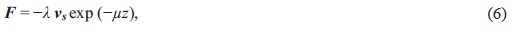where λ = μCDU0 is the inverse of the spindown time scale and vS = (uS, vS) is the surface wind. The quantity CD 10-3 is the drag coefficient, U0 is a characteristic velocity, and μ-1 is roughly the depth over which surface friction acts. If U0 = 5 m s-1 and μ = (500 m)-1, then λ = 10-5 s-1 which is about one third of the typical tropical Coriolis parameter.

The quantity M is the mass source associated with moist convection. In this formulation, the vertical velocity w represents the vertical motion in the environment outside of convection. Convection is represented as a phenomenon which extracts mass from one level and deposits it at a higher level. By mass conservation, in a bounded domain the vertical integral of M must be zero.

Let us now consider the spindown of an initial set of alternating jets in the λ direction, with a kinematic pressure perturbation initially independent of height of the form πG exp(ily), where l is the wavenumber of the jet structure in the y direction. A schematic of the assumed flow is shown in Figure 1. Substitution of the assumed y dependence exp(ily) into (1)-(5) and a bit of algebra yield an equation for the time tendency of pressure: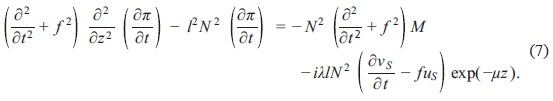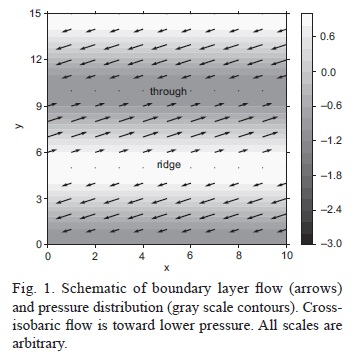We solve this equation subject to the initial condition that π(z) = πG at all levels and assume that ∂π / ∂t, uS, and vS decay exponentially with time dependence exp(-σt). (Note that n itself has a more complex time dependence, which will be explored shortly). Substituting this form into (7) results in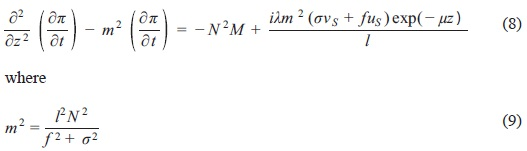is the inverse square of the vertical penetration depth of frictional effects into the free atmosphere.

Since by hypothesis, uS, and vS decay exponentially to zero with time, (2) (3) can be evaluated at the surface as follows:Solving these for uS and vS in terms of the surface pressure perturbation πS results inWe now set M = 0, considering first the convection-free solution to (13). This can be written as the sum of inhomogeneous and homogeneous parts: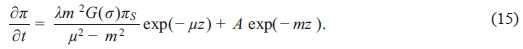The coefficient σ of the homogeneous part is determined by assuming zero buoyancy perturbation at the surface, which implies that ∂π / ∂z there, yielding A = - λμmG(σ)πS / (μ2 - m2), whence the full solution becomesEvaluating this at z = 0 and assuming that πS exp(-σt), we obtain the dispersion relation yielding σ:This equation is implicit, but if λ, σ <<ƒ, then G ≈ 1, and we have σ ≈ λm / (m + μ). Note than in this approximation, a ≤ λ so if λ <<ƒ, as is marginally satisfied for a typical tropical boundary layer with not-too-strong winds, then σ <<ƒ as well. Thus, G ≈ 1 is a reasonable approximation in normal tropical conditions at latitudes exceeding 10° - 15°. Closer to the equator, a more careful evaluation of (17) must be made. (As the unapproximated dispersion relation (17) is cubic in σ, there must be two additional solutions. By plotting the left side of (17) versus the right side as a function of σ for a variety of numerical values of ƒ and λ, it is evident that there is only one real solution of (17). Thus, the remaining two solutions must be complex. We do not consider those solutions here).

Finally, we integrate (16) over the interval [0, t] assume that π = πG at t = 0 and invoke (17) to obtain the kinematic pressure perturbation as a function of time and height:where we have used the geostrophic balance condition ƒ uG = -ilπG to eliminate πG. The x velocity is too complex to write explicitly, but can be obtained from (3), (18), and (21):We now compare the cross-isobaric wind predicted by our model with the simple, steady state wind vSTEADY obtained by setting ∂u /∂t = 0 in (2). For simplicity we continue to assume that λ,σ <<ƒ, which results in significant simplification of the results but does not change their essence. Combining (2) and (6), we find at z; t = 0 that vSTEADY = λuS / ƒ ≈ XuG / ƒ, where we have used ust) = uG exp(-σt). From (17) and (21) and setting G(σ) = 1, the actual cross-isobaric wind at z, t = 0 is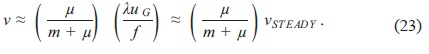Two limits are evident in this result. If μ >>m, then v ≈ vSTEADY, and the actual cross-isobaric wind at the surface is well approximated by the steady-state result. This occurs when the layer in which surface stresses are deposited is much shallower than the resulting penetration depth of momentum fluxes m-1 ≈ ƒ / (IN). In the other limit in which μ <<m, v is less than vSTEADY by the factor μ/ (m + μ).

In the first case, the initial jets spin down in the layer below z ≈ m-1 with significant cross-isobaric flow. This flow results in vertical velocities which lift low-level air underneath cyclonic vorticity regions aloft, and cause air to descend underneath anti-cyclonic regions, in agreement with Charney's ideas cited in section 1. In the second case, insignificant cross isobaric flow occurs, and the jets just spin down in place below z ≈ μ≈-1.

As an example, let us assume that l = 10-5 m, which corresponds to jet widths of about 300 km. Taking typical tropical values of ƒ = 3 x 10-5 s-1 and N = 10-2 s-1, then m-1 = 300 m. For μ-1 = 1500 m, as may occur in a region of trade wind clouds, then μ / (m + μ) = 1/6, and the cross-isobaric flow is highly suppressed. In order for μ/ (m + μ) > 2/3 in this case, then l -1 > 1000 km and jet widths would have to exceed 3000 km. Thus, for typical trade wind jets with horizontal dimensions less than of order 1000 km, the magnitude of cross-isobaric flow and the associated convergence and divergence are much smaller than computed from the steady-state approximation.

Vertical velocities are correspondingly small. Eliminating μG in favor of uG in (20) and setting G(σ) = 1 in (17), we find for t = 0 thatFigure 2 shows the vertical velocity scaled by λuG / N and evaluated at z = μ 1,as a function of m/μ. Since m ∝ l, larger values of m correspond to smaller jet widths.

For weak stratification m/μ << 1. In this case the term in absolute value brackets becomes 1 -exp(-1) and w* is a linear function of m/μ. The dashed line in Figure 2 shows the scaled vertical velocity in this weak stratification limit extrapolated to strong stratifications. Comparison of the solid to the dashed line thus shows how the suppressive effect of stratification on the vertical velocity increases with m/μ.

3. Stratified troposphere topping boundary layer

The analysis in section 2 assumes an infinitely thin boundary layer, which is arguably adequate. In the event that it is not, then a more complex model is called for, one which incorporates a frictional neutral boundary layer topped by a stably stratified layer free of friction. Such a model is developed in this section.

Figure 3 illustrates the ambient structure of the two-layer atmosphere. The neutrally stable boundary layer has an ambient thickness of h and satisfies modified shallow water equations in linearized form. Assuming horizontal structure exp (ily) for all variables as in section 2 and time evolution for the velocity components given by exp(-σt), we have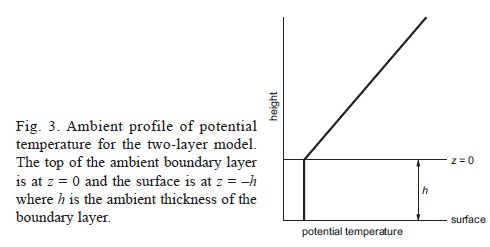where (uB, vB) is the boundary layer wind, the actual boundary layer depth is h(1 + η), πB is the kinematic pressure in the boundary layer at z = 0, MB is the mass source in the boundary layer, and all other symbols are as in section 2. The time derivative of η is retained in (26) as we anticipate a temporal structure for η more complex than simple exponential decay, in analogy with that exhibited by π in section 2. Combining (26)-(28) to eliminate uB and vB, we findFor the normal shallow water equations πB = ghη, but the presence of the overlying stratified atmosphere alters the equation for πB in a manner that we now describe. We assume continuity in potential temperature across the interface between the boundary layer and the free troposphere. This is so because the interface itself is an isentropic surface, so differential advection between the boundary layer and the free troposphere cannot result in potential temperature discontinuities. Since the interface occurs at the elevation zI = , we can express this condition aswhere we have assumed that the buoyancy of boundary layer air bB is uniformly zero in space and time. Expanding b() in a Taylor series about z = 0 and invoking the hydrostatic relation in the free troposphere, we findwhere (∂b / ∂z) hη is ignored because it is nonlinear in the small quantities b and η. The pressure derivative term refers to conditions in the free troposphere.

Ignoring friction and mass sources in the free troposphere, (13) simplifies to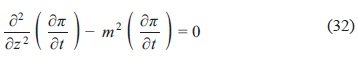where m2 is defined in (9). Based on the experience of section 2, we assume that the pressure in the jet spindown problem takes the formwhere the constant πG has the same meaning as in that section and πX is to be determined by the interface condition (31). The assumed form of π satisfies (32) trivially. From this equation we infer that πB(t) = π(0, t) = πG - [1 - exp(-σt)] πλ and that (∂π / ∂z)z=0 = m [1- exp(-σt)] πX. Eliminating πX between these conditions results inwhich tells us that πB = πG when η = 0. Substitution into (29) provides us with the governing equation for η:Simplifying immediately to the case in which λ, σ <<ƒ and MB = 0, m = IN / ƒ and (35) reduces toUsing a solution method similar to that used for π in section 2, we findsubject to the dispersion relation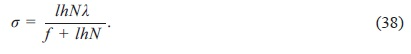The cross-isobaric flow in the boundary layer is obtained from (27):since the cross-isobaric flow in the steady case is vB_STEADY = (λ /ƒ)uB. Thus, the actual cross-isobaric flow is much less than the steady-state cross-isobaric flow if l- <<hN / ƒ . For h = 600 m, N = 10-2 s-1, and ƒ = 3 x 10-5 s-1, hN / ƒ = 200 km. For jet widths much less than about three times this value, the decaying jet lacks significant cross-isobaric flow.

The vertical velocity at the top of the boundary layer is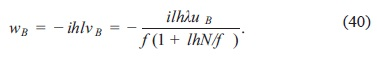As in section 2, we define a dimensionless version of this vertical velocity aswhich is a function only of the dimensionless wavenumber, lhN / ƒ Without the suppressing effects of stratification, we would simply have w* = lhNFigure 4 shows w* as a function of lhN / ƒ in each of these cases. Suppression by stratification is less extreme than in the uniformly stratified case shown in Figure 2, but it is nevertheless significant.4. Frictional convergence and convection

Let us now ask what profile of convective mass source (MS) is required to maintain a steady state in the uniform stratification scenario of section 2. Setting ∂π /∂t = 0 in (13) results in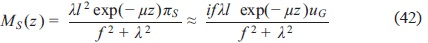where we have used the approximation that πS ≈ πG = ifuG / /. In deriving this expression we have taken σ = 0 in agreement with the steady state assumption, which yields G = ƒ2 / (ƒ2 + λ2) and m2 = l2 N2/ƒ2. This form of MS exhibits a non-zero vertical integral, and is thus not possible by itself; it must be part of a net MS profile M=MS + MF which does satisfy this condition. In regions of cyclonic vorticity and negative πS, the z integral of MS must be negative, which means that the z integral of MF must be positive. A general result arising from these considerations is that no configuration of jets and convection can attain a true steady state in this model.

Another consequence of (42) is that the steady-state MS increases as λ increases until it equals ƒ and decreases thereafter. This is consistent with the numerical results of Montgomery et al. (2010) for intensifying tropical cyclones. Alternatively, as one approaches the equator with fixed λ and uG, the mass flux MS increases, reaches a maximum where ƒ = λ and then decreases.

Similar arguments can be made for the boundary layer topped by a stratified free troposphere, as discussed in section 3, except that the boundary layer mass source required for steady state isCharney and Eliassen (1964) argued in essence that the depth of the free tropospheric secondary circulation associated with cross-isobaric frictional flow is comparable to the depth of the troposphere. As noted in section 1, the steady-state approximation would be valid if this were true, and the vertical motion at the top of the boundary layer could be said to be caused by the action of surface friction. However, as we have shown, typical values of atmospheric stratification result in a far shallower secondary circulation in response to surface friction. Under these circumstances, the time tendency of the wind along the isobars cannot be neglected for a flow of any reasonable horizontal scale (say < 1000 km) in the tropics. For horizontal scales of order 100 km or less, the vertical scale of the secondary circulation is typically much smaller than the thickness of the layer over which surface friction is deposited, especially when there is widespread shallow, moist convection such as occurs in the trade wind regions. In this situation there is essentially no cross-isobaric transport in a boundary layer spinning down under the influence of friction. If some mechanism external to boundary layer dynamics (such as mass sources imposed by deep convection) acts to maintain the boundary layer flow in a nearly steady state, then the time tendency of the boundary layer wind along the isobars becomes small or zero and cross-isobaric flow and resulting convergence exists more or less in the amount predicted by steady-state boundary layer theory. However, the immediate cause of this convergence and the resulting vertical motion at the top of the boundary layer is actually the convection itself, and not boundary layer dynamics; surface friction could be completely absent and the convergence would still exist if the convection were still present.

As discussed in section 1, many investigators postulate that frictional convergence plays an important role in forcing convection in the tropics. However, the suppression of frictional convergence for smaller scales (less than several hundred kilometers in the present context) suggests that this assumption needs to be re-examined. Holton's investigations (Holton et al., 1971; Holton, 1974) demonstrate that friction can have interesting effects on tropical disturbances without invoking the assumption of frictional convergence, i. e., without neglecting the time derivative in the boundary layer momentum equation.

An alternate way of looking at the interaction of convection and frictional convergence might be to imagine that the net effect of the convection is to reduce the effective static stability of the atmosphere, thus resulting in a deeper secondary circulation. However, if the reduction of the static stability is the only effect of the convection, the system would still spin down, albeit more slowly. This is easily ascertained by noting the effects of reducing N2 in the analysis of section 2. For the boundary layer flow to spin up, boundary convergence in excess of that produced by frictional convergence alone is needed. Thus, modeling the effects of moist convection as a simple reduction in static stability does not adequately represent the rich behavior of this phenomenon. For example, if convection acted this way, tropical cyclones would never intensify.

5. Conclusions

We have demonstrated that the stratification of the atmosphere strongly suppresses frictional convergence for typical tropical atmospheric disturbances with scales less than several hundred kilometers. By "typical", we mean disturbances with perturbation velocities of order 5 m s-1. This suppression occurs because the stratification causes the free tropospheric secondary circulation to be much shallower than would be expected in the unstratified case. As a consequence, only a thin layer aloft spins down in response to the secondary circulation as the surface friction dissipates energy. The spindown is therefore more rapid, and the time tendency of the along-isobar component of the horizontal wind in the momentum equation cannot be neglected. This result is demonstrated here for both an atmosphere of uniform static stability and a neutral boundary layer topped by a stably stratified free troposphere.

Charney and Elaissen's (1964) scale analysis of frictional convergence assumes a deep secondary circulation and fails for this reason in the case of weak boundary layer flows. In the limit of a small-scale disturbance, the primary balance in the component of the momentum equation along the isobars is between this time tendency term and friction, not between friction and the Coriolis term. The result is much weaker cross-isobaric flow than would normally be expected when the disturbance is less than a critical size, which is of order several hundred kilometers in our analysis.

In the case of a deep secondary circulation such as envisioned by Charney and Eliassen (1964), the spindown of a disturbance is much slower and the time tendency of the along-isobar component of the wind can be neglected in the momentum equation. The cross-isobaric flow and resulting frictional convergence is close to that obtained from time-independent arguments in this case. If in addition, the frictional convergence produces sufficient ascent to reduce convective inhibition, then the resulting convection can be said to be "caused" by the frictional convergence. When the effects of stratification are included, the situation becomes much more complex. One could imagine that convection located in the regions of boundary layer convergence could boost the strength of this convergence, perhaps supplying enough energy to maintain the boundary layer in a near-steady state. However, even though this convergence is equivalent in magnitude to that produced spontaneously by a boundary layer associated with a deep secondary circulation, one can no longer say that the boundary layer convergence "causes" the convection. In fact, the opposite is true, the convection actually causes the convergence in this case. The convergence cannot have caused the convection, because the convergence would not have been there prior to the convection. The origin of the convection must be sought in some other mechanism.

The attribution of causality in the shallow and deep secondary circulation cases is subtle, but important from the point of view of the parameterization of cumulus convection. Parameterizations in which convection is controlled by frictional convergence computed by a steady-state boundary layer model get the arrow of causality wrong, at least for disturbances small enough to exhibit shallow secondary circulations. This is likely to produce incorrect results in many cases. The solution to this problem is two-fold: (1) include the time derivatives in the boundary layer momentum equations, and (2) drive convection in the model via the mechanisms that are actually responsible for producing it. With these changes, the issue of causality resolves itself.

One tempting shortcut to fixing the problem of shallow secondary circulations is to attempt to represent the effects of deep, moist convection as a simple reduction in the effective static stability, with a corresponding increase in the depth of the secondary circulation. This quick fix has the problem that the resulting model of convection is not nearly rich enough to represent what convection actually does in nature, and is therefore seriously deficient.

Two issues could potentially lead to some alteration of these conclusions. The first is a mathematical point; the analysis performed here is linear. The manifestation of nonlinear effects could be important. Of particular interest is the possibility that advection of vorticity by the convergent flow in the boundary layer could lead to a phenomenon similar to frontal collapse, with the convection resulting from the intense convergence along the front. The geostrophic momentum approximation and semi-geostrophic formalism (Hoskins, 1975) could perhaps be used to tackle this problem.

Raymond et al. (2006) found that an Ekman balance model (pressure gradient + Coriolis force + friction = 0) accurately predicted the meridional wind, and hence meridional convergence, on a day with quiescent conditions in the east Pacific ITCZ. On this day a strong convergent region associated with shallow ascent to 800 hPa occurred near 4° - 6° N, suggesting a front-like structure. Though there was no deep convection occurring in this convergence zone, there certainly must have been ample shallow convection. On a day exhibiting strong, deep convection, large pressure perturbations associated with the convection itself existed in the boundary layer and there was no hint of Ekman balance. This suggests that instances of frictionally driven frontal collapse are delicate and occur only in convectively quiescent conditions. As soon as significant deep convection occurs, more robust mechanisms are likely to dominate.

The second issue has to do with the range of environmental conditions assumed in the present model. Tropical storms have far stronger winds and environmental vorticity. This can result in much deeper and stronger secondary circulations, even on relatively small scales, thus paving the way for significant frictional convergence in the boundary layer. The tropical storm case deserves an independent analysis of the effects of stratification on frictional convergence.

Acknowledgments

This work was supported by National Science Foundation grant ATM-1021049 and Office of Naval Research grant N000140810241.

References

Charney J. G. and A. Eliassen, 1949. A numerical method for predicting the perturbations of the middle-latitude westerlies. Tellus 1, 38-54.         [ Links ]

Charney J. G. and A. Eliassen, 1964. On the growth of the hurricane depression. J. Atmos. Sci. 21, 68-75.         [ Links ]

Charney J., 1971. Tropical cyclogenesis and the formation of the intertropical convergence zone. In: Lectures in Applied Mathematics. American Mathematical Society 355-368.         [ Links ]

Charney J., 1973. Movable CISK. J. Atmos. Sci. 30, 50-52.         [ Links ]

Duck P. W. and M. R. Foster, 2001. Spin-up of homogeneous and stratified fluids. Ann. Rev. Fluid. Mech. 33, 231-263.         [ Links ]

Ekman, V. W. 1905. On the influence of the earth's rotation on ocean-currents. Arkiv F. Matem, Astr, o Fysik (Stockholm), Band 2, No. 11, 1-53.         [ Links ]

Ekman V. W. 1906. Beitráge zur Theorie der Meeresstrõmungen. Ann. Hydrograph. Marit. Meteorol. 2, 1-50.         [ Links ]

Greenspan H. P. and L. N. Howard, 1963. On a time-dependent motion of a rotating fluid. J. Fluid Mech. 17, 385-404.         [ Links ]

Holton J. R., J. M. Wallace and J. A. Young, 1971. On boundary layer dynamics and the ITCZ. J. Atmos. Sci. 28, 275-280.         [ Links ]

Holton, J. R., 1974. On the influence of boundary layer friction on mixed Rossby-gravity waves. Tellus 27, 107-115.         [ Links ]

Holton J. R., 1965. The influence of viscous boundary layers on transient motions in a stratified rotating fluid. Part I. J. Atmos. Sci. 22, 402-411.         [ Links ]

Holton J. R. and P. H. Stone, 1968. A note on the spin-up of a stratified fluid. J. FluidMech. 33, 127-129.         [ Links ]

Hoskins B. J., 1975. The geostrophic momentum approximation and the semi-geostrophic equations. J. Atmos. Sci. 32, 233-243.         [ Links ]

Montgomery M. T., R. K. Smith and S. V. Nguyen, 2010. Sensitivity of tropical-cyclone models to the surface drag coefficient. Q. J. Roy. Meteor. Soc. 136, 1945-1953.         [ Links ]

Ooyama K., 1969. Numerical simulation of the life cycle of tropical cyclones. J. Atmos. Sci. 26, 3-40.         [ Links ]

Pedlosky J., 1967. The spin up of a stratified fluid. J. Fluid Mech. 28, 463-479.         [ Links ]

Raymond D. J., C. S. Bretherton and J. Molinari, 2006. Dynamics of the intertropical convergence zone of the east Pacific. J. Atmos. Sci. 63, 582-597.         [ Links ]

Sakurai T., 1969. Spin down problem of rotating stratified fluid in thermally insulated circular cylinders. J. Fluid Mech. 37, 689-699.         [ Links ]

Wang B., 1988. Dynamics of tropical low-frequency waves: An analysis of the moist Kelvin wave. J. Atmos. Sci. 45, 2051-2065.         [ Links ]

Wang B. and H. Rui, 1990. Dynamics of the coupled moist Kelvin-Rossby wave on an equatorial beta plane. J. Atmos. Sci. 47, 397-413.         [ Links ]All the contents of this journal, except where otherwise noted, is licensed under a Creative Commons Attribution License